[BACK]
 Computers, Materials & ContinuaDOI:10.32604/cmc.2021.017666Article

PAPR Reduction in NOMA by Using Hybrid Algorithms

Department of Electronics and Communication Engineering, JECRC University, Jaipur, 303905, India
*Corresponding Author: Arun Kumar. Email: arun.kumar1986@live.com
Received: 06 February 2021; Accepted: 02 April 2021

Abstract: Non-orthogonal multiple access (NOMA) is gaining considerable attention due to its features, such as low out-of-band radiation, signal detection capability, high spectrum gain, fast data rate, and massive D2D connectivity. It may be considered for 5G networks. However, the high peak-to-average power ratio (PAPR) is viewed as a significant disadvantage of a NOMA waveform, and it weakens the quality of signals and the throughput of the scheme. In this article, we introduce a modified NOMA system by employing a block of wavelet transform, an alternative to FFT (Fast Fourier transform). The modified system combines the details of fractional frequency and time analysis of NOMA signals. In this correspondence, we utilize an advanced partial transmission scheme (PTS), and selective mapping (SLM), and present a genetic algorithm (GA) for SLM to investigate the peak power performance of a WT-based NOMA system. The performance of WT-SLM, WT-PTS, and WT-SLM-GA methods is compared with that of the traditional NOMA-based SLM and PTS methods. The simulation results demonstrate that the proposed system effectively reduces PAPR in comparison with the traditional schemes.

Keywords: PAPR; wavelet transform; NOMA; PTS; SLM; 5G

1  Introduction

2  System Model

2.1 Wavelet Transform (WT)

This study investigates the performance of dynamic NOMA signals in time and frequency domains. WT is identical to Fourier transform (FT). In FT, the function is restrained in the Fourier space. By contrast, WT utilizes the function restricted in the Fourier and real-time span. The abilities to calculate fast and to study sub-details of signals are considered significant benefits of WT. It is utilized to split signals into the elements of a wavelet. Hence, it is feasible to acquire an excellent estimation of the function by utilizing a small number of coefficients, compared with FT. However, high-cost implementation, utilization of massive numbers of WT, distortion in signals, and lengthy compacting time are few of the drawbacks of WT. Mathematically, WT can be expressed as :

Ψn+1,2m1[k]=2k¯h[k¯]Ψn,m[k2nk¯](1)

Ψn+1,2m1[k]=2k¯g[k¯]Ψn,m[k2nk¯](2)

where h[k] and g[k] are the characteristics of high and low-pass filters, representing the wavelet and scalar functions; Ψn,m is the mth wavelet function on the nth level. The IDWT of the transmitted signal can be represented as:

y=IDWT(Y)(3)

y(t)=m=0N=1l=0y(l)Ψn,msy(tlN)(4)

where y(l) is the transmitted signal, m indicates the position of l signal index, Ψn,msy represents the wavelet function of m-channel, and N is the number of subcarriers.

2.2 Estimation of the PAPR of the NOMA Waveform

The schematic of the wavelet-based NOMA waveform is indicated in Fig. 1. In this proposed model, IDWT and DWT blocks are introduced in place of FFT and IFFT. The proposed system allows the access of each subcarrier by all users.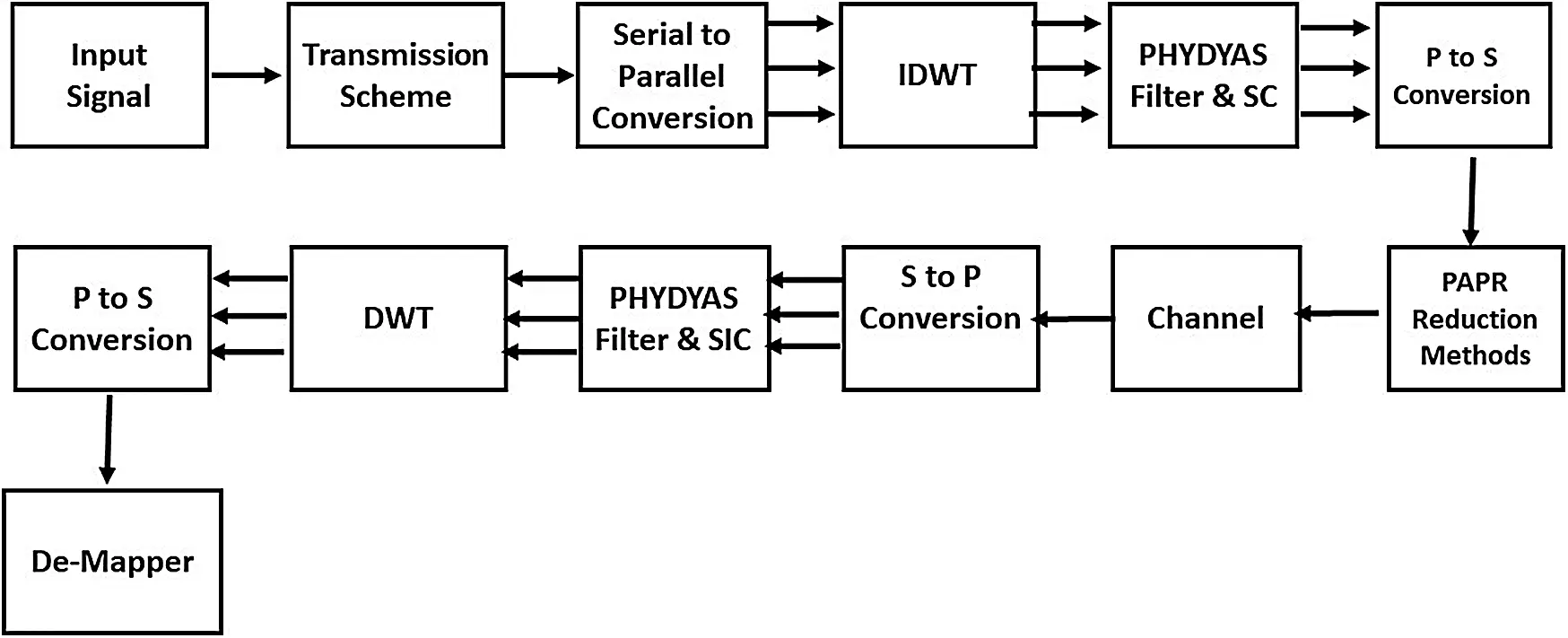Figure 1: Schematic of NOMA

The NOMA signal is given as:

Yk=0.707n=0N1x(n)expj2πknN(5)

where n=0,1,2,,N1. The IDWT of Eq. (1) is given as:

y=IDWT(Yk)(6)

y(t)=m=0N=1l=0yk(l)Ψn,msy(tlN)(7)

IDWT converts the NOMA signal into a time-domain NOMA signal. A PHYDAS filter is applied to the time-domain NOMA signal, which is given as:

Y(t)=expj2πfctn=0N1y(t)h(L)(8)

h(L) is the characteristic of the PHYDAS filter, which is given as:

h(L)=1+2K=1K1(1k)hkcos(6.28kmkL)(9)

Eq. (8) can be written as:

Y(t)=expj2πfctn=0N1y(t)1+2K=1K1(1k)hkcos(6.28kmkL)(10)

fc is the carrier frequency and L is the size of the PHYDYAS filter. The PAPR of NOMA is expressed as:

PAPRY(t)=MaximumtT|Y(t)|21T0T|Y(t)|2dt,

where T is the period of the NOMA symbol. Traditionally, PAPR is expressed in terms of dB, given as:

PAPRY(t)dB=10log10MaximumtT|Y(t)|21T0T|Y(t)|2dt(11)

The complementary cumulated distribution function (CCDF) is a significant parameter which indicates the effectiveness of reduction methods. Hence, PAPR should be defined in terms of the CCDF of NOMA, as shown as follows:

CCDF(PAPRY(t))=Pr(PAPRY(t)>PAPRo). For more than one number of antennas, it can be expressed as:

Pr(PAPRY(t)>PAPRo)=1(1expPAPR0)MtN(12)

MtN denotes the NOMA signal in the time domain.

2.3 Wavelet Transform Based SLM (SLM-WT)

The SLM technique was presented in 1996 . SLM is regarded as one of the most efficient PAPR minimization methods, as shown in Fig. 2. The purpose of the conventional SLM, is to generate an optimal phase vector for the sub-blocks of the NOMA symbol and IFFT are applied to it. A low-PAPR signal is then chosen and transmitted. However, SLM introduces a high computational complication due to the use of IFFTs. In the projected system, the optimal phase factor is multiplied with NOMA symbols, and the IDWT block is used instead of IFFT. The NOMA signal is applied to IDWT, which converts the NOMA signal into a time-domain one. Lastly, a low-peak-power signal is selected and transmitted with low computational complexity.Figure 2: DWT-SLM

The NOMA symbols are divided into several sub-blocks given as:

Z=[Z0, Z1, , ZN1](13)

The phase vector (Pv) is provided as:

Pv=P0v, P1v ,, PNv1(14)

where v=1, 2, , V and Pv is the permitted phase alteration. The sub-blocks of NOMA symbols (Z) and phase vector Pv are multiplied, resulting in:

Zv(k)=Pv(k)Z(k)(15)

Zv(k)=[P0vZ(0), P1vZ(1), , PN1vZ(N1]T(16)

K=0, 1, 2,,N1. The IDWT of Eq. (16) is given as:

znv=IDWT(Zv(k))(17)

znv=IDWT(Pv(k)Z(k))(18)

znv(t)=m=0N=1l=0Zv(k)Ψn,msy(tlN)(19)

znv(t)=m=0N=1l=0[P0vZ(0), P1vZ(1),,PN1vZ(N1]TΨn,msy(tlN)(20)

A low-PAPR NOMA signal is given as:

PAPRznv(t)=MaximumtT|znv(t)|21T0Tznv(t)2dt(21)

PAPR is expressed in terms of dB, given as:

PAPRznv(t)dB=10log10MaximumtT|znv(t)|21T0Tznv(t)2dt(22)

From Eq. (22), the proposed SLM-DT method depends on V and N. Thus, varying V and N results in the optimal reduction of PAPR.

2.4 Wavelet Transform Based PTS (PTS-WT)

The PTS method was first implemented in 1997 . In this method, the NOMA symbols are divided into several data blocks applied to IDWT. The data blocks are weighted using a phase element (W) to produce a low-value PAPR signal. The proposed model is shown in Fig. 3.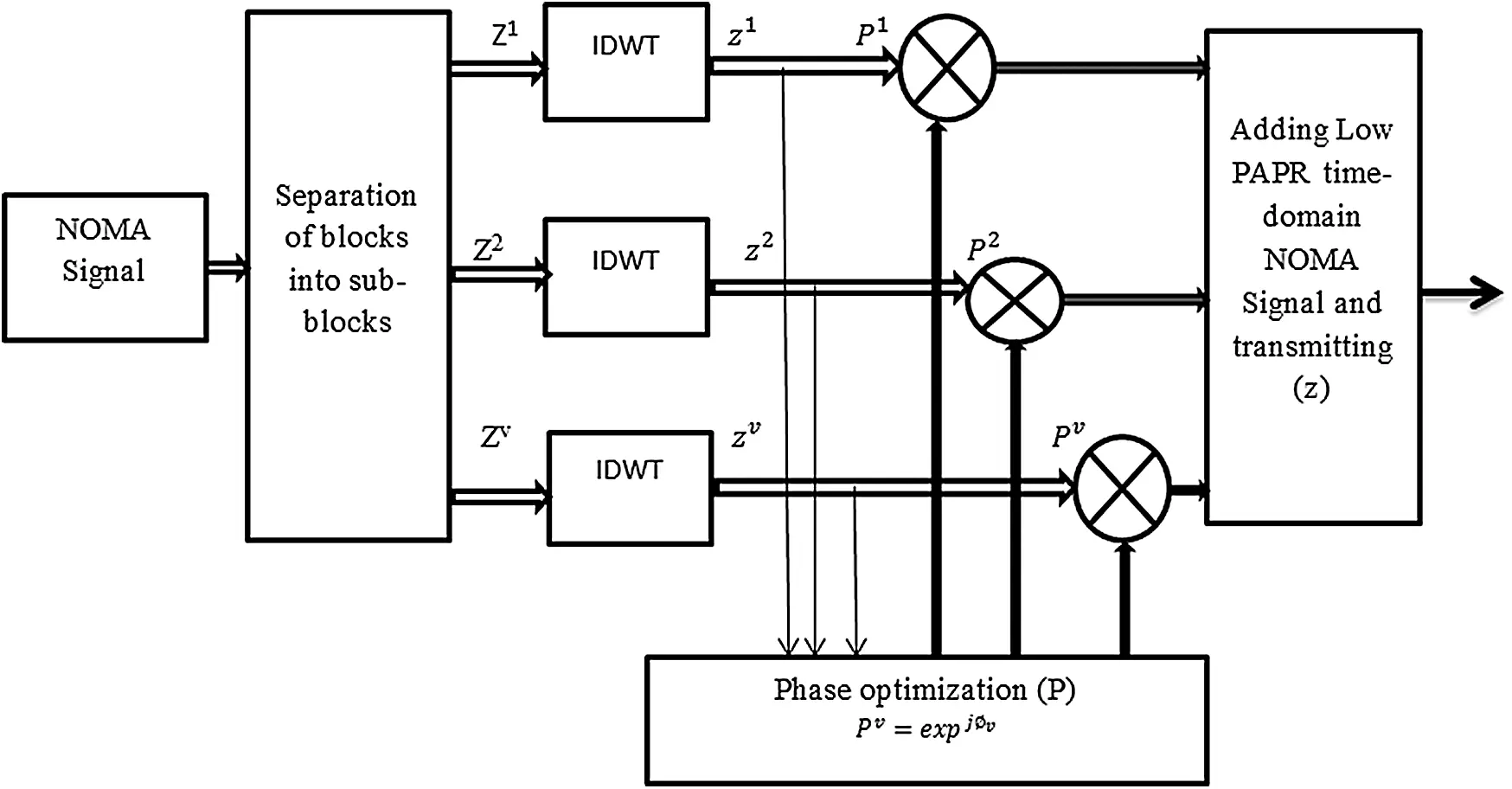Figure 3: WT-PTS

The NOMA symbols are given as Z=[Z0, Z1, , ZN1], divided into several numbers of sub-blocks (v), expressed as:

Z=[Z0, Z1, , Zv](23)

The sub-blocks are orthogonal to one another. Hence, the NOMA symbol (Z) can be expressed as the addition of entire sub-blocks V, given as:

Z=v=1VZv (18). The IDWT of Eq. (23) is:

zv=IDWT(Zv(k))(24)

zv(t)=m=0N=1l=0Zv(k)Ψn,msy(tlN)(25)

The phase factor is given as:

Pv=expjv, where 0,2π. The primary objective is to generate the best phase factor for which minimum peak power is obtained. Vector Pv is expressed as:

P=[P1, P2, , Pv]T(26)

A minimum-PAPR NOMA signal is achieved by multiplying Eqs. (25) and (26), as shown as follows:

z(t)=m=0N=1l=0Zv(k)Ψn,msy(tlN)[P1, P2, , Pv]T(27)

The phase alteration factor is limited toPv=expj6.28lw, l=0, 1, 2, , W, W represents the permitted phase factors. The PAPR of PTS-WT-based NOMA is expressed as:

PAPRz(t)=MaximumtTz(t)21T0Tz(t)2dt(28)

PAPR is expressed in terms of dB, given as:

PAPRz(t)dB=10log10MaximumtTz(t)21T0Tz(t)2dt(29)

2.5 Wavelet Transform Based SLM-GA (SLM-GA-WT)

We present a hybrid PAPR method grounded in artificial intelligence centered genetic algorithm sustained, SLM sequence denoted as SLM-GA, to reduce the peak power of NOMA. In the hybrid method, peak power minimization is accomplished and complexity is significantly reduced. NOMA signals are divided into different sub-blocks and applied to IDFT converting frequency-domain NOMA sub-blocks in time-domain NOMA sub-blocks. GA is introduced to generate the best phase rotation factor and added to NOMA data blocks. Further, the GA also reduces the complexity of the NOMA waveform. The WT-GA-SLM system is depicted in Fig. 4. The NOMA signal is denoted as:

Z=[Z0, Z1, , ZN1]T(30)

The phase factor is generated using GA, given as:

PGAu=[P0u, P1u, , PNu]T(31)

PGAuv=expiuv(32)

The NOMA data symbol is given as:

Zv=[Z0v, Z1v, , ZN1v]T(33)

The NOMA symbols (Zv) and phase vector PGAuv are multiplied, resulting in:

Z=ZvPGAuv(34)

Z=[Z0v, Z1v, , ZN1v]Texpiuv(35)

The IDWT of Eq. (28) is expressed as:

z(t)=IDWT(Z)(36)

z(t)=m=0N=1l=0Z(k)Ψn,msy(tlN)(37)

The low-PAPR signal is determined as:

PAPRz(t)=MaximumtTz(t)21T0Tz(t)2dt(38)

PAPR is expressed in terms of dB, given as:

PAPRz(t)dB=10log10MaximumtTz(t)21T0Tz(t)2dt(39)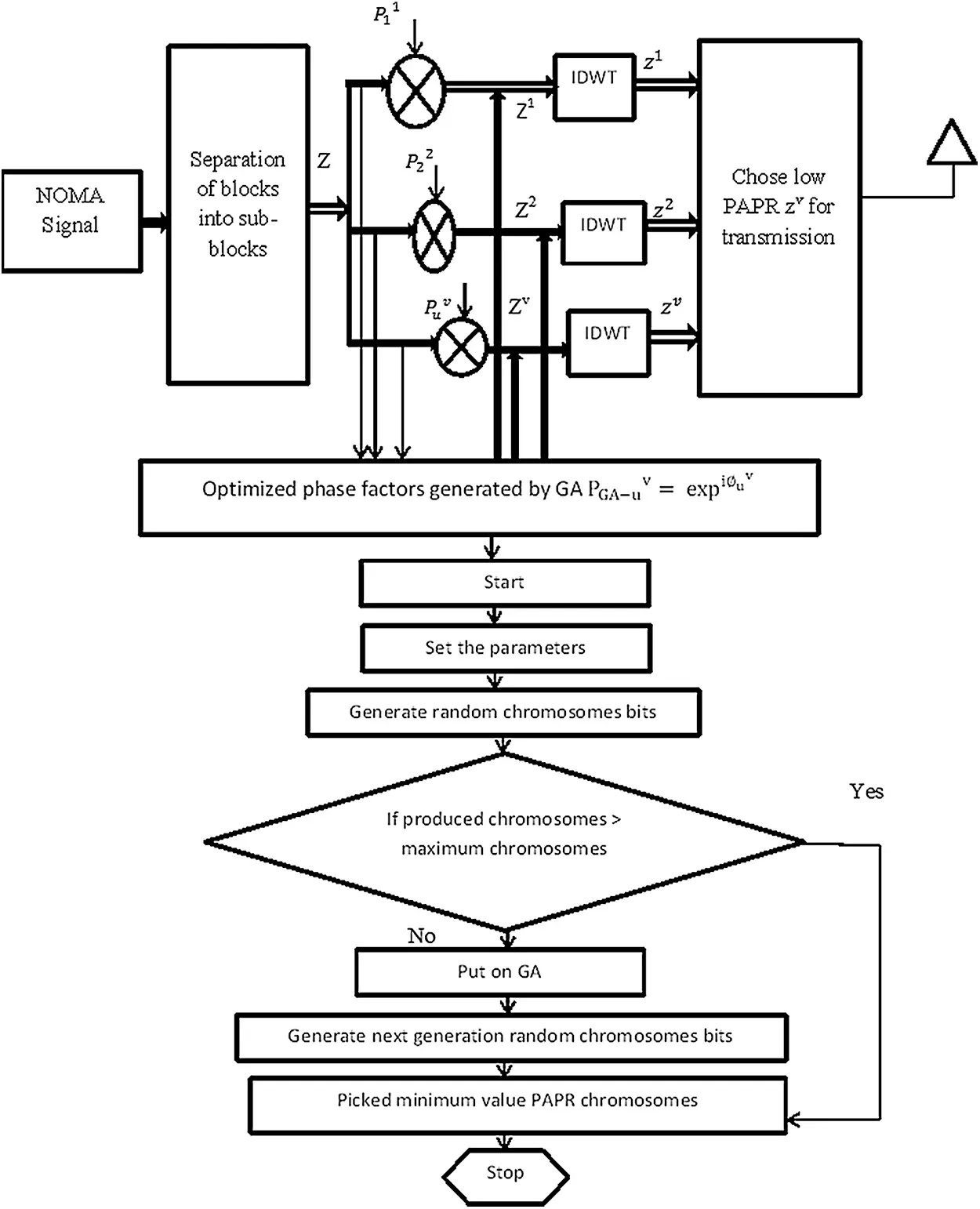Figure 4: WT-GA-SLM

3  Simulation Results

In this work, we employ MATLAB-2014 to assess the performance of the projected and old-style approaches [23,24]. The parameters of the proposed simulation are indicated in Tab. 1.

Table 1: Simulation parameters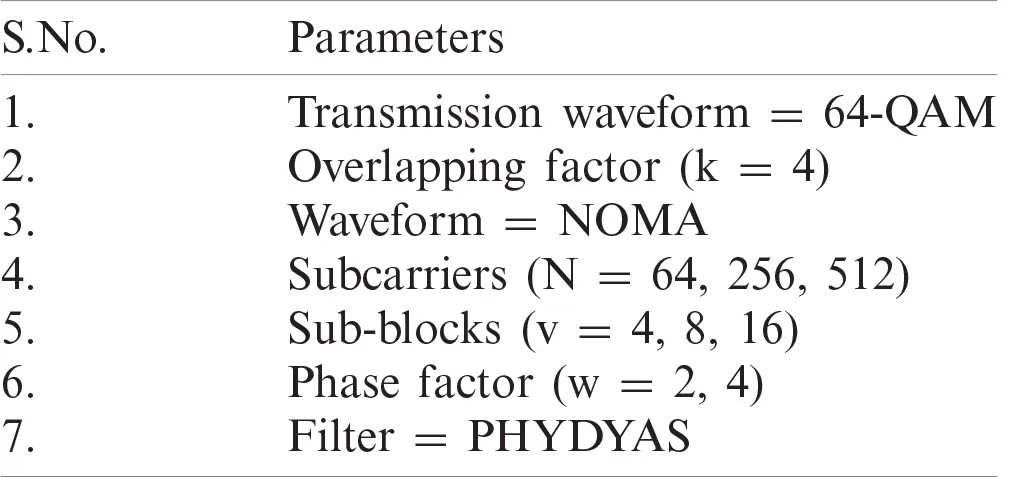Fig. 5 represents the peak power performance of the NOMA waveform for N number of subcarriers used in the simulation (N = 64, 256, and 512). N = 64, 256, 512 sub-carriers minimize the amplitude power to 8.5, 9.2, and 14 dB respectively, at the CCDF of 10−3. Hence, we can obtain optimal PAPR performance by utilizing N = 64.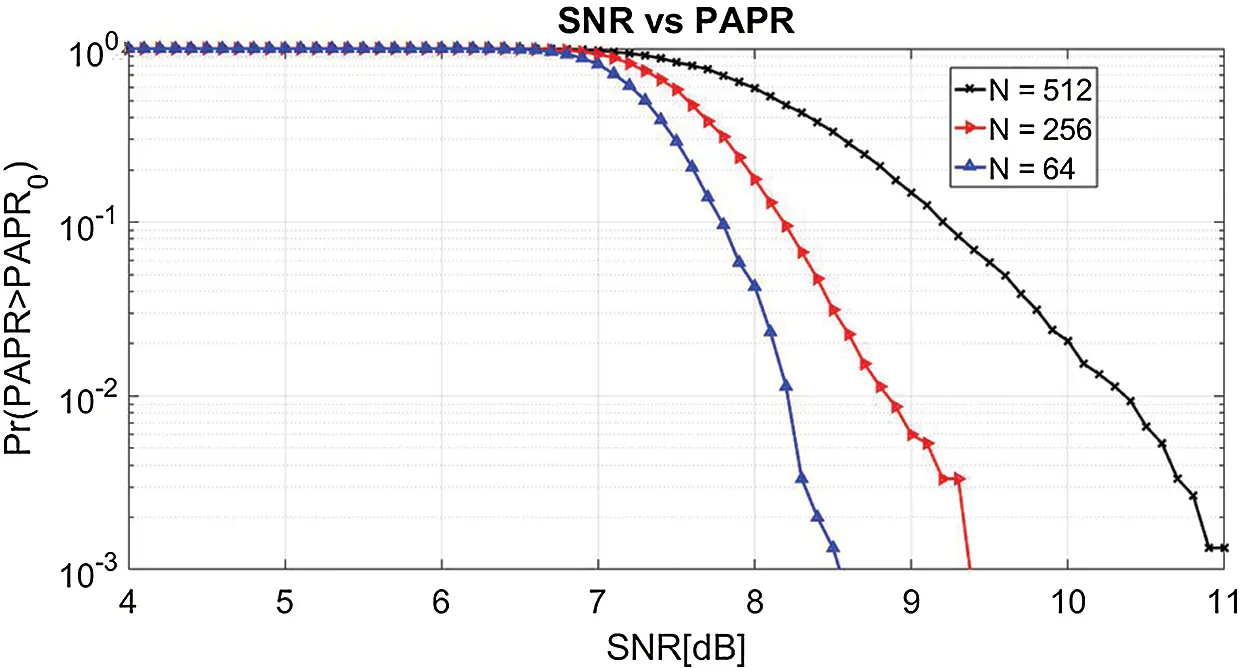Figure 5: PAPR for different sub-carriers (N)

In Fig. 6, we analyze the peak power reduction capability of the proposed WT-NOMA and FFT-NOMA systems. The simulation results demonstrate that the proposed WT-NOMA significantly reduces the PAPR to 9.8 dB compared with the traditional NOMA (12 dB).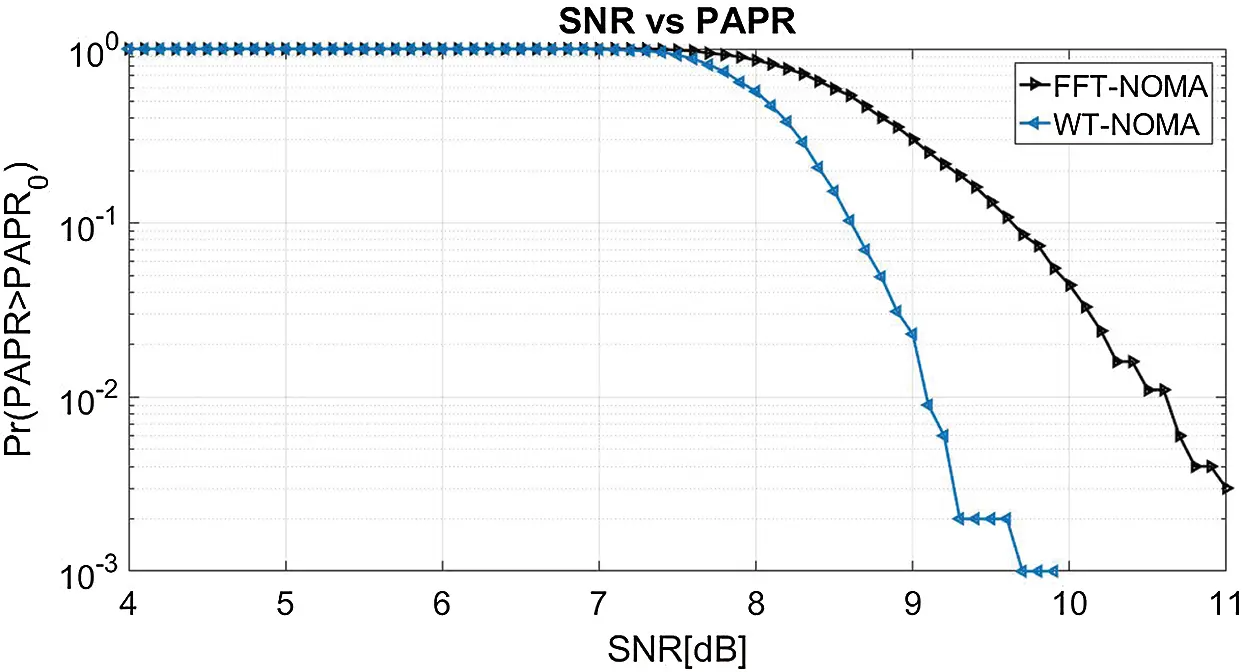Figure 6: PAPR performance of WT-NOMA vs. FFT-NOMA

The peak power analysis of the proposed WT-SLM and FFT-SLM for the NOMA system is presented in Fig. 7. The original PAPR of NOMA at the CCDF of 10−3 is 11 dB. From the simulation results, the proposed method reduces the peak power to 8.7 dB whereas the conventional SLM shows peak power of 9.8 dB. Accordingly, the proposed method achieves a gain of 1.26 dB compared with to the FFT-SLM.Figure 7: PAPR characteristics

The PAPR analysis of DT-SLM for different sub-blocks (v) is shown in Fig. 8. In the present simulation, v is restricted to 4, 8, and 16 data blocks. The original PAPR of NOMA at the CCDF of 10−3 is 11 dB. The results indicate that the proposed WT-SLM reduces the peak power to 9, 8.2, and 7.4 dB for v = 4, 8, 16 respectively. As projected, the amplitude power of the NOMA signal can be minimized by varying the value of v. From the results, ideal PAPR can be realized using v = 16 in WT-SLM.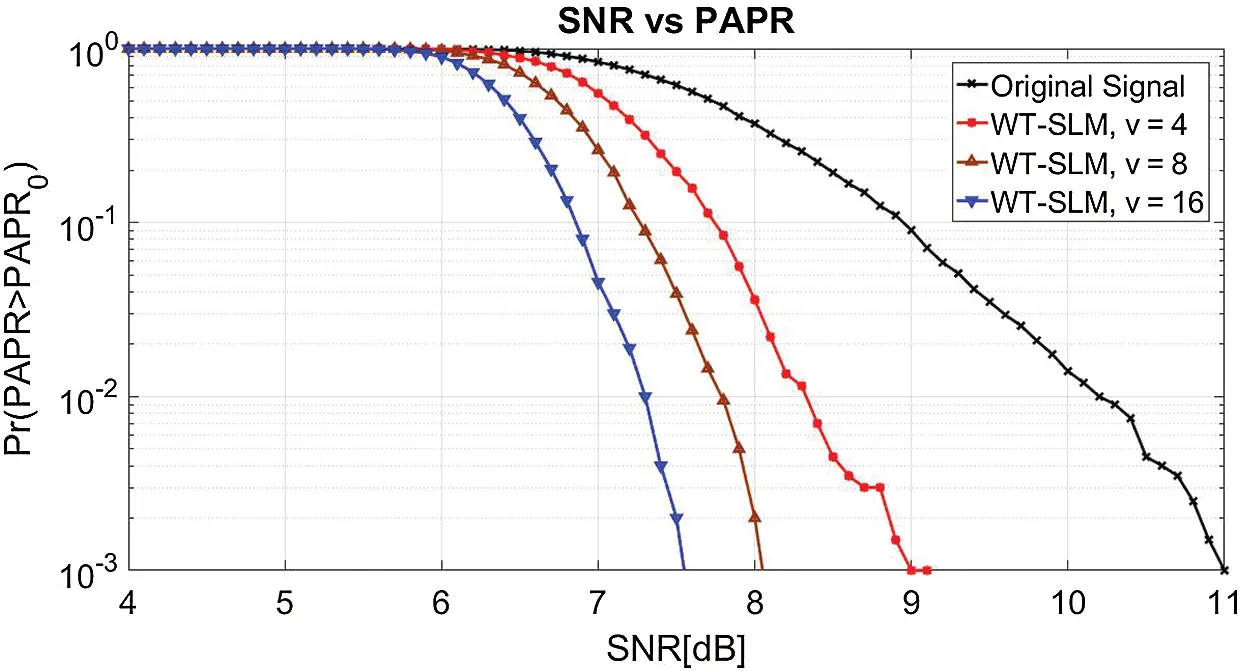Figure 8: PAPR of WT-SLM with v

In Fig. 9, we investigate the PAPR performance of the proposed WT-PTS and FFT-PTS at CCDF of 10−3. The results show that the proposed method efficiently reduces the amplitude power to 7 dB, whereas the traditional PTS has peak power of 9.4 dB. Hence, WT-SLM has better performance than the traditional PTS.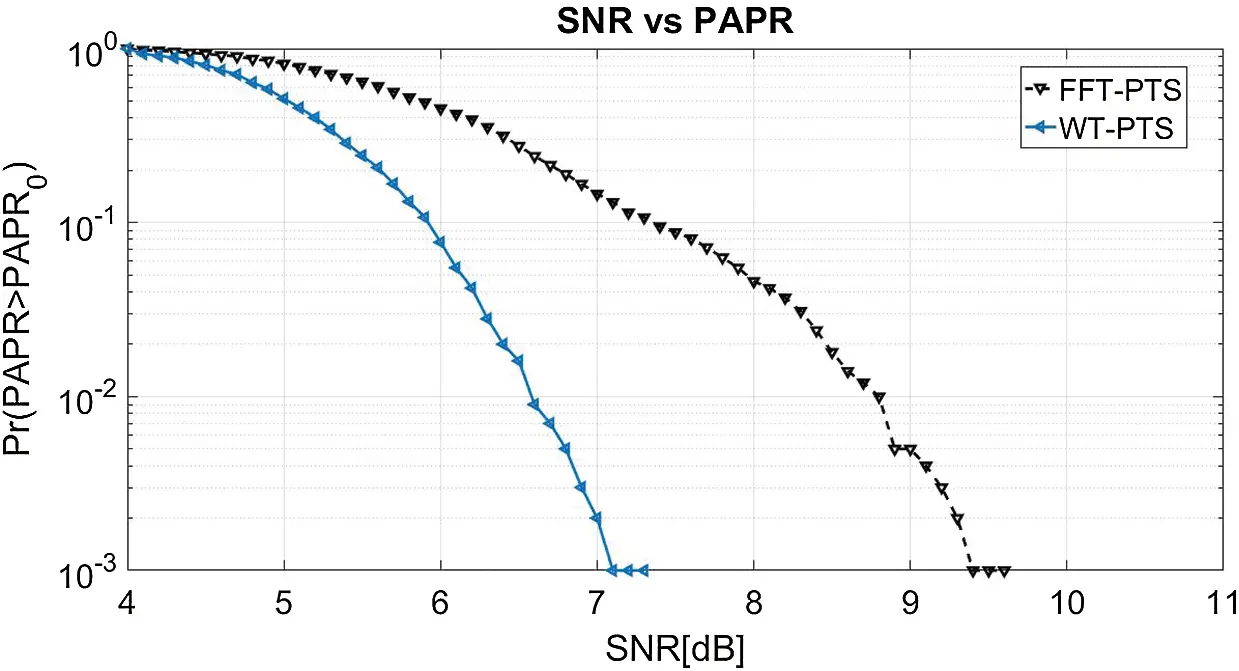Figure 9: PAPR for WT-PTS and FFT-PTS

The PAPR curve of the recommended WT-PTS for the NOMA wave is indicated in Fig. 10. We confine our simulation outcomes to repetition values v and w equivalent to 2. As estimated, enhanced peak power reduction is realized by changing the values of u and w. At the CCDF of 10-3, WT-PTS minimizes the peak power to 4.2, 5, 6.8, and 8.6 dB for v = 4 w = 4, v = 4 w = 2, v = 2 w = 4, and v = 2 w = 2, respectively, compared with the original PAPR (11 dB). From the curve, optimal PAPR performance can be achieved using v = 4 w = 4 in WT-PTS.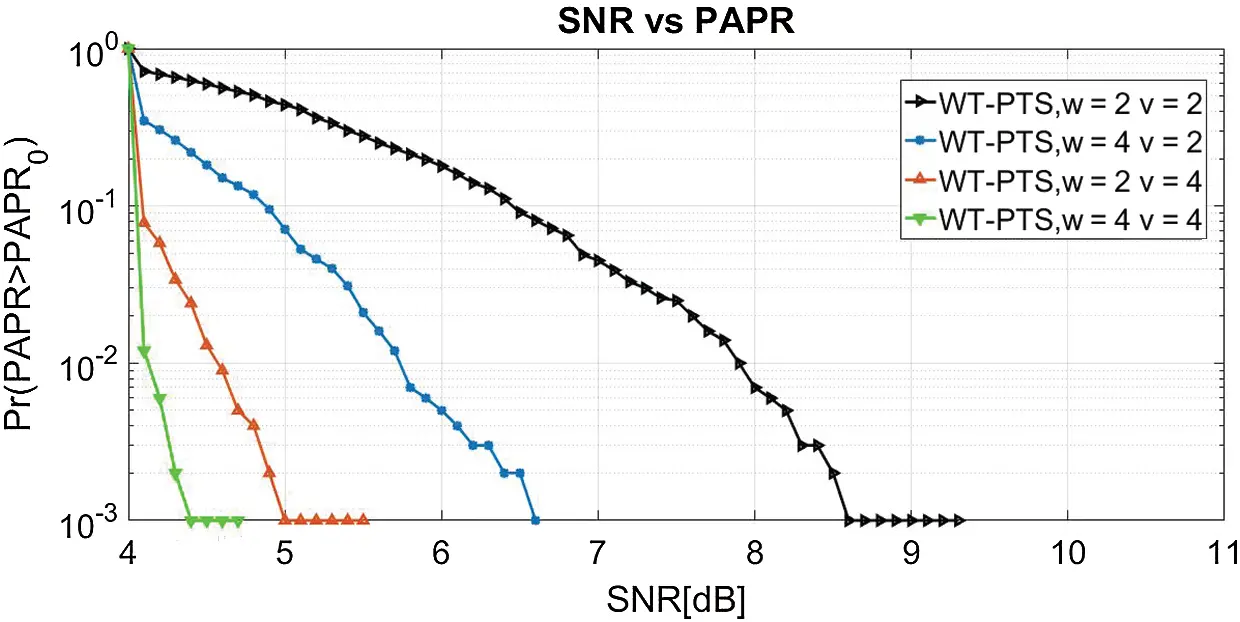Figure 10: PAPR of WT-PTS with v and w

In the NOMA waveform, the PAPR curve of the proposed SLM-GA is achieved with varying ‘u’, and ‘v’ numbers, as indicated in Fig. 11. The original peak power of the NOMA signal without the reduction method is 11 dB. At the CCDF of 10−3, the peak power is reduced to 4.1 and 5.8 dB for WT-SLM-GA w = 4 and w = 2, respectively. Thus, efficient PAPR performance is obtained using w=4 in WT-SLM-GA.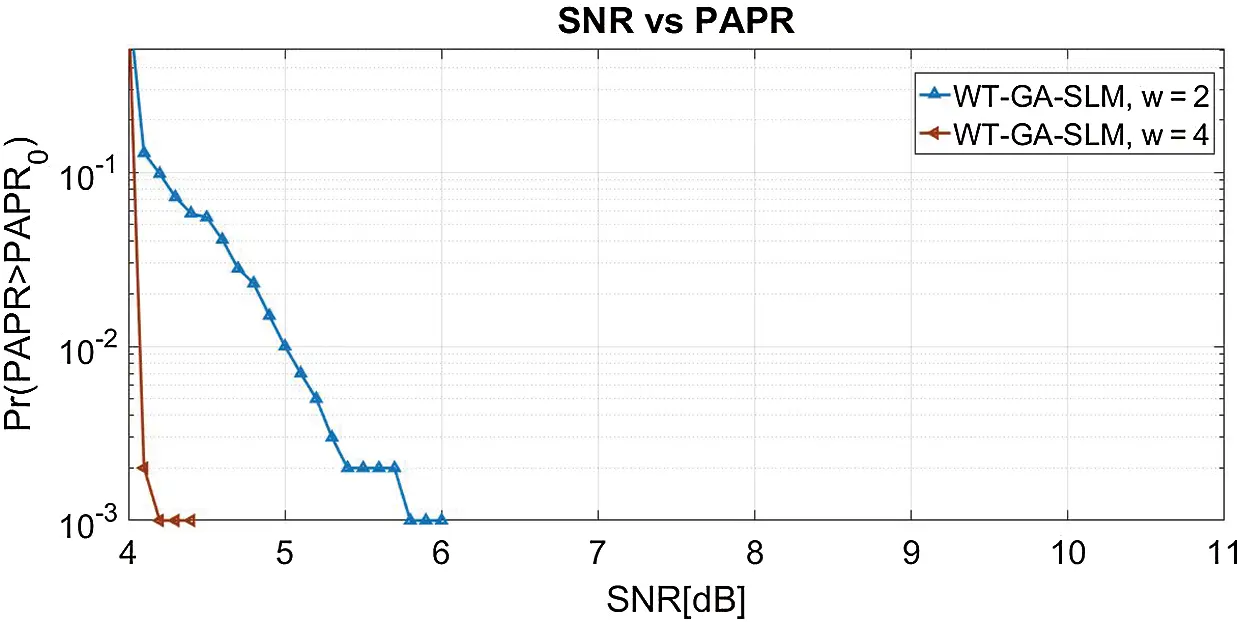Figure 11: PAPR of WT-GA-SLM with w

In Fig. 12, we compare the performance of the BER curve when the signal is applied to FFT-NOMA and WT-NOMA. From the simulation results, the BER of FFT-NOMA is 10−3 at 7.8 dB SNR. When the signal is passed through WT-NOMA, the BER of 10-3 is obtained at the SNR of 5.3 dB. Hence, WT-NOMA provides better results than FFT-NOMA.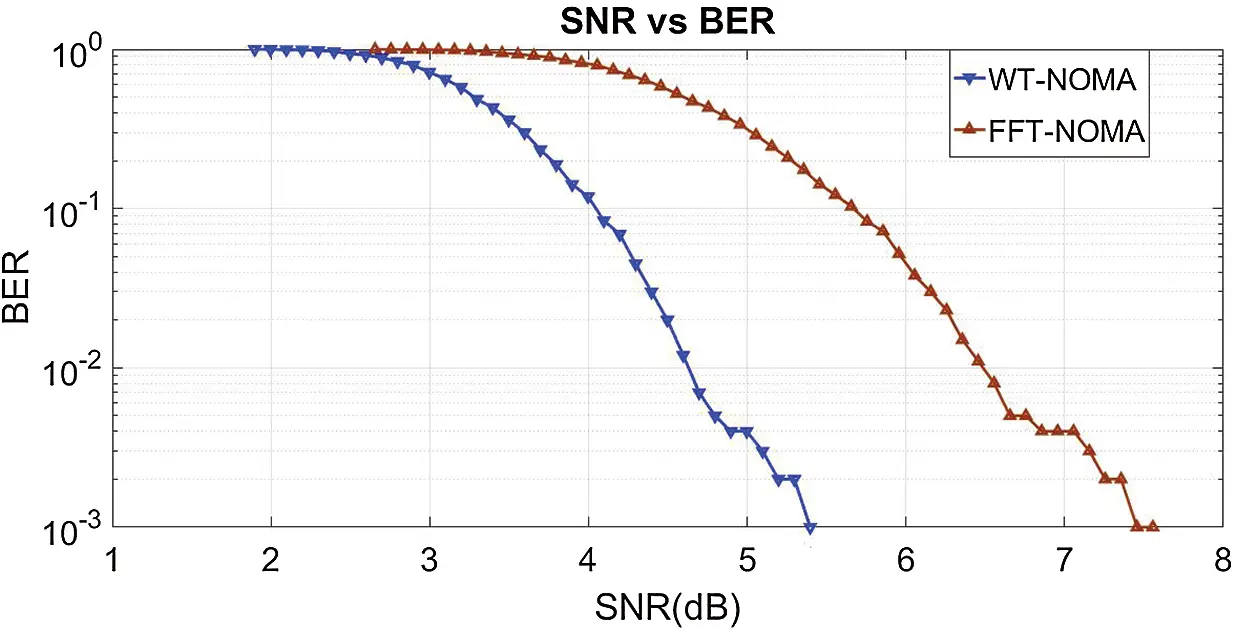Figure 12: BER of FFT-NOMA and WT-NOMA

To analyze the PAPR performance in NOMA, the SNR verses BER characteristic curves are plotted for the proposed and conventional reduction schemes, in Fig. 13. BER of 10−3 is obtained at SNRs of 4.2 dB for WT-SLM-GA w = 4, 6.8 dB for WT-PTS v = 4, w = 4, 6.2 dB for WT-SLM v = 16, 8.4 dB for FFT-SLM, and 10.2 dB for FFT-PTS. Therefore, WT-SLM-GA outperforms other reduction schemes.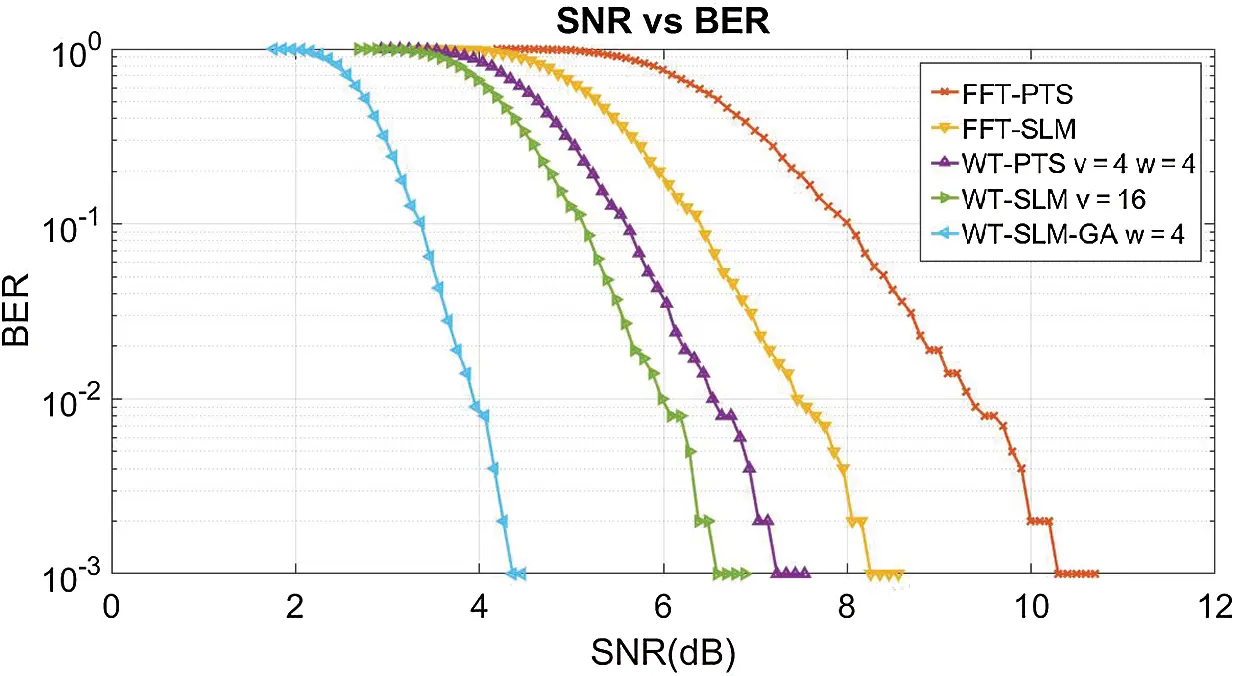Figure 13: BER curve

Tabs. 2 and 3 indicate the gain and power saving obtained by the reduction methods, respectively.

Table 2: Gain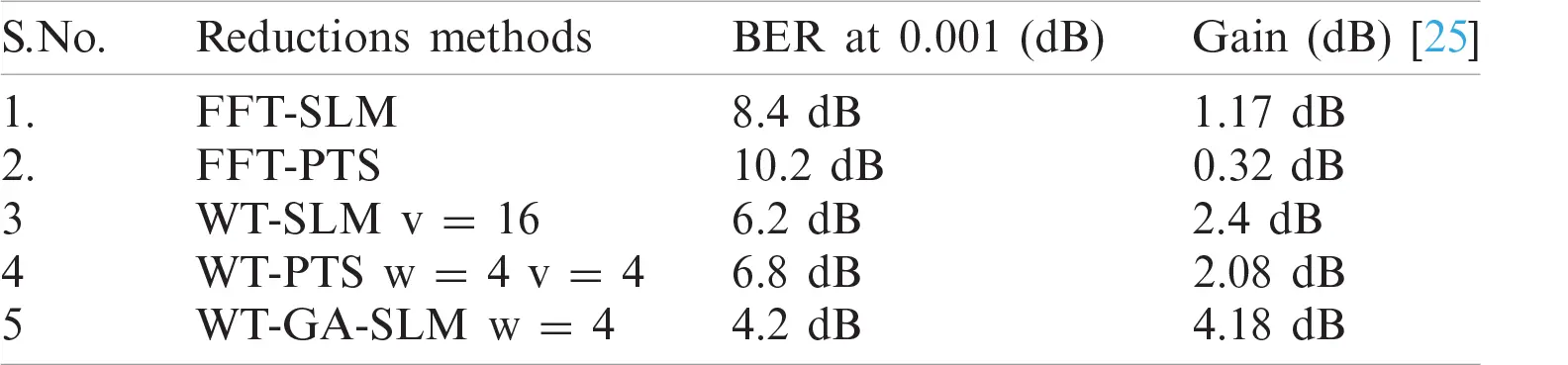Table 3: Power saving of reduction approaches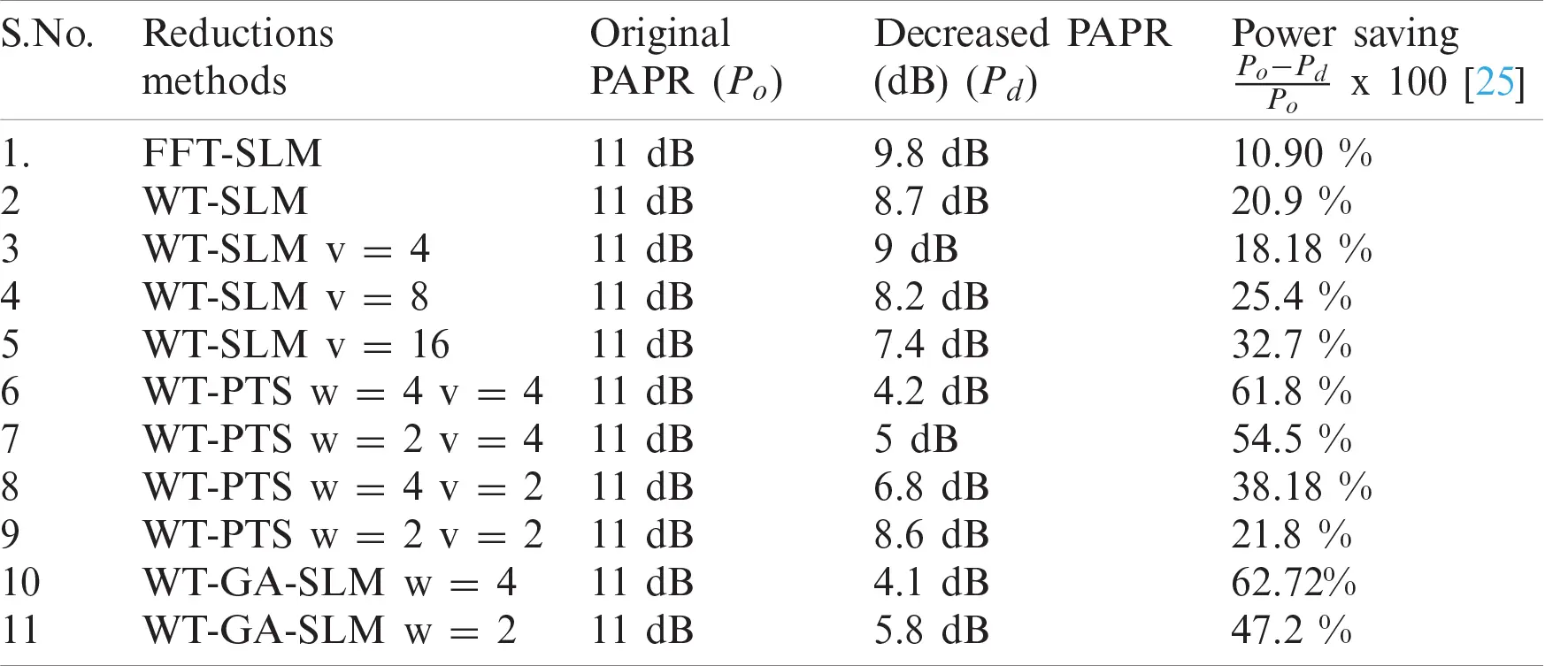4  Conclusion

We propose a novel peak power reduction method for the NOMA waveform. For the first time, WT blocks are introduced into the NOMA structure. Time-domain NOMA signals are generated and processed by applying inverse WT to acquire a fair amount of sub-blocks with a minimal number of IFFTs. Hence, NOMA-based WT (WT-NOMA) gains better PAPR and BER than the conventional NOMA (FFT-NOMA). The peak power of the transmitted WT-NOMA signals is studied by modifying the PTS and SLM techniques and SLM and PTS techniques increases the complexity of the WT-NOMA system. Therefore, we introduce a GA-based SLM method for the WT-NOMA waveform. Moreover, the proposed WT-centered reduction methods are compared with conventional SLM, PTS, and NOMA (FFT-NOMA). From the simulation analysis, WT-SLM v = 16, WT-PTS v = 4 w = 4, and WT-GA-SLM w = 4, obtain the best performance. The amplitude power is reduced to 7.4, 4.2, and 4.1 dB compared with that of the original PAPR (11 dB). The conventional SLM, PTS and FFT-NOMA systems are outperformed. We also plot the BER curve to investigate the performance of the best proposed methods and traditional methods. The outcomes demonstrate that the BER performance of WT-GA-SLM better than that of SLM v = 16, PTS w = 4 v = 4, FFT-SLM, and FFT-NOMA.

Acknowledgement: The authors would like to thank the editors of CMC and the anonymous reviewers for their time in reviewing this manuscript. The authors also acknowledge JECRC University for providing a lab facility.

Funding Statement: The authors received no specific funding for this study.

Conflicts of Interest: The authors declare that they have no conflicts of interest to report regarding the present study.

## References

1. A. Kumar and M. Gupta, “A novel modulation technique for 5G mobile communication system,” American Journal of Applied Sciences, vol. 12, no. 9, pp. 602–605, 2015.
2. X. Zhang, L. Chen, J. Qiu and J. Abdoli, “On the waveform for 5G,” IEEE Communications Magazine, vol. 54, no. 11, pp. 74–80, 2016.
3. G. Kongara, C. He, L. Yang and J. Armstrong, “A comparison of CP-OFDM, PCC-OFDM and UFMC for 5G uplink communications,” IEEE Access, vol. 7, pp. 157574–157594, 2019.
4. G. Liu, Z. Wang, J. Hu, Z. Ding and P. Fan, “Cooperative NOMA broadcasting/multicasting for low-latency and high-reliability 5G cellular V2X communications,” IEEE Internet of Things Journal, vol. 6, no. 5, pp. 7828–7838, 2019.
5. Y. Saito, Y. Kishiyama, A. Benjebbour, T. Nakamura, A. Li et al., “Non-orthogonal multiple access (NOMA) for cellular future radio access,” in 2013 IEEE 77th Vehicular Technology Conf. (VTC SpringDresden, pp. 1–5, 2013.
6. K. Tahkoubit, A. Ali-Pacha, H. Shaiek and D. Roviras, “Iterative dichotomy PAPR reduction method for multicarrier waveforms,” IEEE Communications Letters, vol. 23, no. 11, pp. 2073–2076, 2019.
7. I. Baig, N. U. Hasan, M. Zghaibeh, I. U. Khan and A. S. Saand, “A DST precoding based uplink NOMA scheme for PAPR reduction in 5G wireless network,” in 7th Int. Conf. on Modeling, Simulation, and Applied Optimization, Sharjah, pp. 1–4, 201
8. I. Baig, “On the PAPR reduction: A novel filtering based Hadamard transform precoded uplink MC-NOMA scheme for 5G cellular networks,” in 1st Int. Conf. on Computer Applications & Information Security, Riyadh, pp. 1–4, 201
9. I. Baig, “A precoding-based multicarrier non-orthogonal multiple access scheme for 5G cellular networks,” IEEE Access, vol. 5, pp. 19233–19238, 2017.
10. A. Kumar and M. Gupta, “Peak average power reduction in NOMA by using PTSCT technique,” Recent Patents on Computer Science, vol. 13, no. 3, pp. 502–507, 2019.
11. M. H. Aghdama and A. A. Sharifib, “PAPR reduction in OFDM systems: An efficient PTS approach based on particle swarm optimization,” ICT Express, vol. 5, no. 3, pp. 178–181, 2019.
12. M. Tören and C. Çiflikli, “Peak-to-average-power-ratio reduction methods with wavelet transform in MIMO-OFDM,” IETE Journal of Research, vol. 64, no. 3, pp. 415–421, 2018.
13. W. Rong, J. Cai and X. Yu, “Low complexity PTS PAPR reduction scheme for UFMC systems,” Cluster Computing, vol. 20, pp. 3427–3440, 2017.
14. H. Rathore and A. Kumar, “Reduction of peak average power ratio for FBMC waveform with P-PTS technique,” International Journal of Sensors, Wireless Communications and Control, vol. 10, no. 1, pp. 47–54, 2020.
15. A. S. Fathy, M. S. E. Mahallawy, S. M. Gasser and E. A. A. Hagras, “PAPR reduction based on genetic algorithm for PTS wavelet-OFDM systems,” in Proc. of the 2019 3rd Int. Conf. on Advances in Artificial Intelligence, New York, NY, USA: Association for Computing Machinery, pp. 58–62, 2019.
16. A. Lahcena, A. Saidab and A. Adela, “Low computational complexity PTS scheme for PAPR reduction of MIMO-OFDM systems,” Procedia Engineering, vol. 181, pp. 876–883, 2017.
17. V. Sudha and D. Sriram Kumar, “Low complexity PAPR reduction in SLM-OFDM system using time domain sequence separation,” Alexandria Engineering Journal, vol. 57, no. 4, pp. 3111–3115, 2018.
18. K. Mhatrea and U. P. Khotb, “Efficient selective mapping PAPR reduction technique,” Procedia Computer Science, vol. 45, pp. 620–627, 2015.
19. A. Kumar, “A novel hybrid PAPR reduction technique for NOMA and FBMC system and its impact in power amplifiers,” IETE Journal of Research, vol. 5, no. 1, pp. 1–17, 20
20. R. Ayeswarya and A. N. Prabha, “Fractional wavelet transform based PAPR reduction schemes in multicarrier modulation system,” IETE Journal of Research, 2019. https://doi.org/10.1080/03772063.2019. 1621685.
21. R. W. Bauml, R. F. Fischer and J. B. Huber, “Reducing the peak-to-average power ratio of multicarrier modulation by selected mapping,” Electronics Letter, vol. 32, no. 22, pp. 2056–2057, 1996.
22. S. H. Muller and J. B. Huber, “OFDM with reduced peak to-average power ratio by optimum combination of partial transmit sequences,” Electronics Letter, vol. 33, no. 5, pp. 368–369, 1997.
23. Y. A. A. Jawhar, N. S. M. Shah, M. A. Taher, M. S. Ahmed and K. N. Ramli, “An enhanced partial transmit sequence segmentation schemes to reduce the PAPR in OFDM systems,” International Journal of Advanced Computer Science and Applications, vol. 7, no. 12, pp. 66–75, 2017.
24. P. P. Kumar and K. K. Kishore, “BER and PAPR analysis of UFMC for 5G communications,” Indian Journal of Science and Technology, vol. 9, no. 1, pp. 1–6, 2016.
25.  F. H. Raab, P. Asbeck, S. Cripps, P. B. Kenington and B. Z. Popovic, et al., “Power amplifier and transmitters for RF and microwave,” IEEE Transactions Microwave Theory and Techniques, vol. 50, no. 3, pp. 814–826, 2002.This work is licensed under a Creative Commons Attribution 4.0 International License, which permits unrestricted use, distribution, and reproduction in any medium, provided the original work is properly cited.